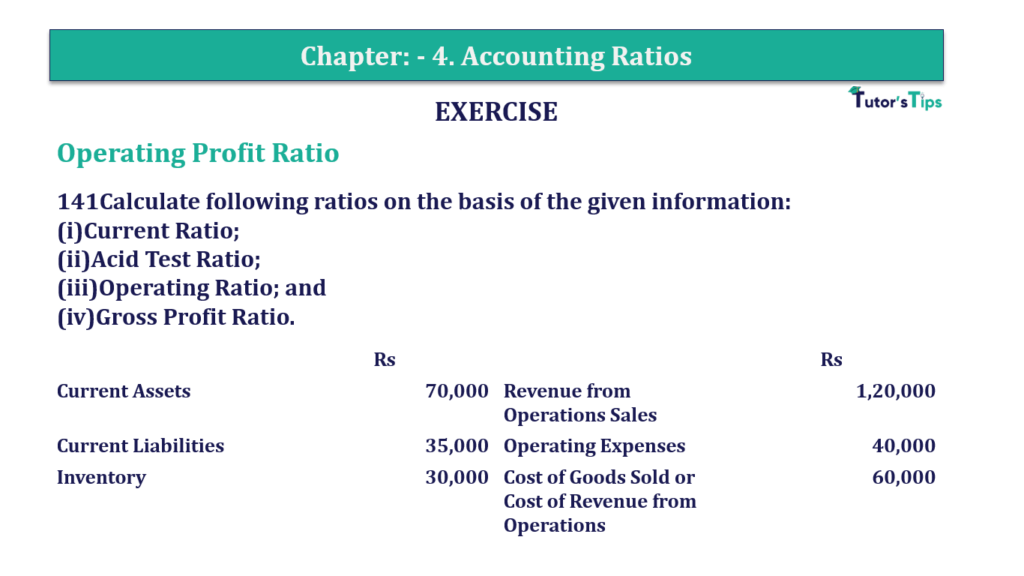# Question 141 Chapter 4 of +2-B – T.S. Grewal 12 ClassQuestion 141 Chapter 4 of +2-B

Miscellaneous

141Calculate following ratios on the basis of the given information:
(i)Current Ratio;
(ii)Acid Test Ratio;
(iii)Operating Ratio; and
(iv)Gross Profit Ratio.

 Rs Rs Current Assets 70,000 Revenue from Operations Sales 1,20,000 Current Liabilities 35,000 Operating Expenses 40,000 Inventory 30,000 Cost of Goods Sold or Cost of Revenue from Operations 60,000

### The solution of Question 141 Chapter 4 of +2-B: –

I

 Current Assets = Rs 70,000 Current Liabilities = Rs 35,000
 Gross Profit Ratio = Current Assets Current Liabilities
 Gross Profit Ratio = Rs. 70,000 Rs. 70,000 = 2 : 1

II

 Liquid Assets = Current Assets − Inventory = Rs 70,000 − Rs 30,000 = Rs 40,000
 Acid Test Ratio = Liquid Assets Current Liabilities
 Acid Test Ratio = Rs. 40,000 Rs 35,000 = 1.14 : 1

III

 Net Sales = Rs 1,20,000 Operating Cost = Cost of Goods Sold + Operating Expenses = Rs 60,000 + Rs 40,000 = Rs 1,00,000
 Operating Ratio = Operating Cost X 100 Net Sales
 Operating Ratio = Rs. 1,00,000 X 100 Rs 1,20,000 = 83.33%

IV

 Gross Profit = Net Sales − Cost of Goods Sold = Rs 1,20,000 – Rs 60,000 = Rs 60,000 Average Inventory = Rs 15,000
 Gross Profit Ratio = Gross Profit X 100 Net Sales
 Gross Profit Ratio = Rs 60,000 X 100 Rs 1,20,000 = 50%

Balance Sheet: Meaning, Format & Examples

Comment if you have any question.

Also, Check out the solved question of previous Chapters: –

### T.S. Grewal’s Double Entry Book Keeping +2 (Vol. I: Accounting for Not-for-Profit Organizations and Partnership Firms)

• Chapter No. 1 – Financial Statement of Not-For-Profit Organisations
• Chapter No. 2 – Accounting for Partnership Firms – Fundamentals
• Chapter No. 3 – Goodwill: Nature and Valuation
• Chapter No. 4 – Change in Profit-Sharing Ratio Among the Existing Partners
• Chapter No. 5 – Admission of a Partner
• Chapter No. 6 – Retirement/Death of a Partner
• Chapter No. 7 – Dissolution of a Partnership Firm

### T.S. Grewal’s Double Entry Book Keeping (Vol. II: Accounting for Companies)

• Chapter No. 1 – Financial Statements of a Company
• Chapter No. 2 – Financial Statement Analysis
• Chapter No. 3 – Tools of Financial Statement Analysis – Comparative Statements and Common- Size Statements
• Chapter No. 4 – Accounting Ratios
• Chapter No. 5 – Cash Flow Statement